Need help? Call us +31 20 40 99 300

## Basic properties

### Weight (ISO 536, TAPPI T410):

 What The basis weight of paper, board or corrugated board in g/m²​ Why A constant weight contributes to consistency. This value is of importance when choices for means of distribution have to be made (mail or otherwise) and is also needed to interpret other physical properties that are specified with regard to weight. How On an analytical balance weighing of 10 samples of 100 cm². Optional: weighing at least 10 samples of known area (e.g. 210×297 mm=A4) and calculating the weight in g/m². Calculations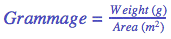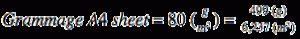Where: m = mass of 1 sample in g A = area of 1 sample in m²Report the average of at least 10 significant values and the standard deviation.

### Bulk (ISO 534, TAPPI T411):

 What This is the ratio between thickness and weight, the specific volume, expressed in cm³/g. A higher value indicates a higher bulk. Why Bulk of paper is e.g. used to determine the total thickness of a book or magazine. How Measuring both weight and thickness as described, calculating the bulk. Calculations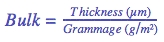Report the average of at least 10 significant values and the standard deviation.

### Mass Density (ISO 534, TAPPI T411):

 What To show how much mass is present in a specific volume, expressed in g/cm³. Why Density has impact on opacity and strength properties. How Measuring both weight and thickness as described, calculating the mass. Calculations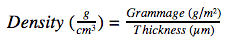Report the average of at least 10 significant values and the standard deviation.

### Thickness (ISO 534, TAPPI T411, ISO 3034):

 What The distance between front and back of a single sheet of paper, board or corrugated board, expressed in µm (1 micrometre = 0.001 mm) Why Variation in thickness causes variation in the printed image and may be of influence on certain finishing processes. How 20 Measurements in micrometres with a conforming thickness gauge. Another way of measuring thickness is to measure the total thickness of a stack of 10 sheets on 5 specified places. Average results divided by 10. This is often referred to as bulking thickness as opposed to single sheet thickness. (Official ISO standard is withdrawn.) Calculations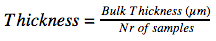Where: ts  = thickness of a single sheet tb = thickness of a stack of 10 sheets (bulking thickness)Report the average of at least 10 significant values and the standard deviation.